# GMAT Math : Calculating whether right triangles are similar

## Example Questions

### Example Question #1 : Triangles

Which set of side lengths cannot be the side lengths of a right triangle?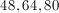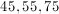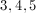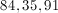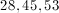Explanation:

For a triangle to be a right triangle, the sides must obey the Pythagorean Theorem. Let's try our options.

3, 4, 5: You should know this is a right triangle without having to do any calculations because it is one of the special triangles that you should remember. But if you didn't,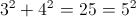.

28, 45, 53: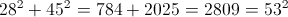45, 55, 75: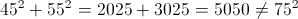. The sides don't follow the Pythagorean Theorem so this can't be a right triangle. This is our answer. Let's check the remaining two sets of sides as well.

48, 64, 80: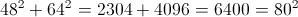. These are pretty big numbers and this math might take a while. Instead of doing these calculations, we could also see if 48, 64, and 80 look like any of the special triangles we know. Let's divide the three numbers by 16. 48/16 = 3, 64/16 = 4, and 80/16 = 5. Then this is just a type of 3,4,5 triangle, which we know is a right triangle.

84, 35, 91: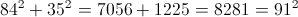. Again, these are big numbers to square. Let's divide the three numbers by their greatest common factor, 7. 84/7 = 12, 35/7 = 5, 91/7 = 13. Then this is a 5, 12, 13 triangle, which is another of our special triangles that we know is a right triangle.

### Example Question #2 : Triangles

Which of the following right triangles is similar to one with a height ofand a base of?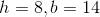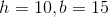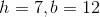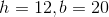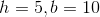Explanation:

In order for two right triangles to be similar, the ratio of their dimensions must be equal. First we can check the ratio of the height to the base for the given triangle, and then we can check each answer choice for the triangle with the same ratio: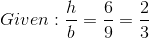So now we can check the ratio of the height to the base for each answer option, in no particular order, and the one with the same ratio as the given triangle will be a triangle that is similar: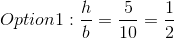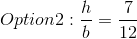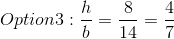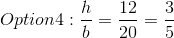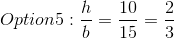The triangle with a height ofand a base ofhas the same ratio as the given triangle, so this one is similar.

### Example Question #1 : Calculating Whether Right Triangles Are Similar

Which of the following right triangles is similar to one with a heightofand a baseof?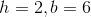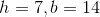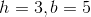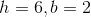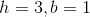Explanation:

In order for two right triangles to be similar, their height-to-base ratios must be equal. Given a right triangle with a height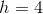and a base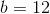, the ratio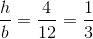. The only answer provided with a ratio ofis### Example Question #4 : Triangles

Which of the following right triangles is similar to one with a heightofand a baseof?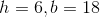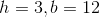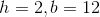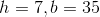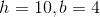Explanation:

In order for two right triangles to be similar, their height-to-base ratios must be equal. Given a right triangle with a height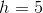and a base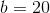, the ratio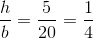. The only answer provided with a ratio ofis.

### Example Question #5 : Triangles

Which of the following right triangles is similar to one with a heightofand a baseof?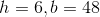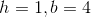None of the above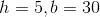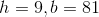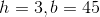Explanation:

In order for two right triangles to be similar, their height-to-base ratios must be equal. Given a right triangle with a heightofand a baseof,  the ratio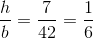. The only answer provided with a ratio ofis.

### Example Question #6 : Triangles

Given two right triangles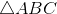and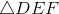, with right angles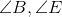, what is the measure of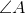?

Statement 1: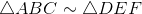Statement 2: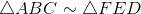BOTH STATEMENTS TOGETHER do NOT provide sufficient information to answer the question.

STATEMENT 2 ALONE provides sufficient information to answer the question, but STATEMENT 1 ALONE does NOT provide sufficient information to answer the question.

STATEMENT 1 ALONE provides sufficient information to answer the question, but STATEMENT 2 ALONE does NOT provide sufficient information to answer the question.

EITHER STATEMENT ALONE provides sufficient information to answer the question.

BOTH STATEMENTS TOGETHER provide sufficient information to answer the question, but NEITHER STATEMENT ALONE provides sufficient information to answer the question.

BOTH STATEMENTS TOGETHER provide sufficient information to answer the question, but NEITHER STATEMENT ALONE provides sufficient information to answer the question.

Explanation:

Corresponding angles of similar triangles are congruent, so Statement 1 alone establishes that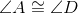and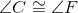; similarly, Statement 2 alone establishes that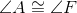and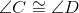. However, neither statement alone establishes the actual measure of any of the four acute angles.

Assume both statements are true. From transitivity, it holds that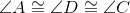. Specifically, the two acute angles of, one of which is, are congruent. Since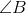is right, this makesand isosceles right triangle, and its acute angles, including, have measure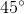Tired of practice problems?

Try live online GMAT prep today.